# A High Frequency Dielectric Behavior Modeling of a ReXTMnO2 Ternary Composite as an Equivalent Binary Mixture

A High Frequency Dielectric Behavior Modeling of a ReXTMnO2 Ternary Composite as an Equivalent Binary Mixture

Abdelhalim BrahimiNacerdine Bourouba Juan Pablo Martinez Jiménez Nacerdine Bouzit

Laboratoire Instrumentation Scientifique LIS, Département d’Electronique, Faculté de Technologie, Université Ferhat Abbas Sétif 1, Sétif 19000, Algeria

Corresponding Author Email:
abdelhalim.brahimi@univ-setif.dz
Page:
181-191
|
DOI:
https://doi.org/10.18280/rcma.310401
5 June 2021
|
Accepted:
9 August 2021
|
Published:
31 August 2021
| Citation

OPEN ACCESS

Abstract:

The goal of this paper is to study the dielectric behavior, in the ternary composites microwave domain, and simplifying ternaries models into binaries ones using time domain spectroscopy. We worked on three ternary composites made of epoxy resin (Re), manganese dioxide (MnO2) and one of three different titanates (XT): barium titanate (BT), calcium titanate (CT) or magnesium titanate (MT). These composites samples dielectric behavior is inspected over a frequency range from DC to 5 GHz. This aims to optimize the predictive modified Lichtenecker's law model (MLL) through a better smoothing shape factor making theoretical and experimental results very close to each other. The comparison between these results shows that the MLL model is pertinent for ternary mixtures (Re-XT-MnO2) with an appreciable accuracy degree. Another numerical approach applied to the Re-[Re-XT-MnO2] mixtures using a binary mixture model equivalent to the previous ternary one based on a simplified MLL expression and preserving the same mixture constituents. This model results are in good agreement not only with ternaries ones being modeled with this law but also with other modeling laws appreciated with binary composites. This study interest lies on these materials application in the telecommunications components miniaturization and in microwave electronic applications.

Keywords:

mixture's laws, modeling, shape factor, ternary, binary

1. Introduction

High Dielectric materials are key materials of many emerging applications and they are called so according to having a permettivity value of dielectric constant kx8.854F/cm more than that of specific materials (k>7). One of many high material advantages achieved, in electronic industry, until now is a rise of passive component number per chip with optimum circuit performance .

Composite materials based on dielectric nano-particles and polymers are widely used in electronics and microwave technology. These materials are very promising for wide range of applications such as optoelectronics, radio absorbing coatings in microwave technology with adaptive absorption and transmission characteristics and also for energy storage [2, 3]. Microwave measurements of materials with high dielectric constants have permitted accurate dielectric characterization and have led to a fine material selection for microwave applications. In particular, ferroelectric materials have aroused a great interest in the gigahertz frequency region and have given rise to several unrelenting researches for their application purpose. Thanks are due to their relatively high dielectric constant and their success being attributed to its excellent ferroelectric and piezoelectric properties [4, 5]. Therefore, ferroelectrics become attractive by their applications in many fields and more particularly in nano-electronics, communication devices, electromechanical systems and sensors .

The dielectric properties of random heterogeneous materials have received more and more attention and this research continues to be of a major interest. To this end, the topical subject focuses on the development of a deeper understanding that leads to innovative technological applications that satisfy specific needs required by their application fields such as electronics, automotive and aerospace, etc. One of the particular concerns of this research cluster is the dielectric permittivity of this material type which is a very complex function because of its dependence on its constituents permittivities, their volume fractions and the inclusions shape. It is noted that this effective permittivity determination is subject to the mixture complexity degree. For a mixture with a unique and periodic structure, the permittivity doesn’t depend on the space variables but it remains constant at all points of the mixture, thus leading to an analytical evaluation of the permittivity by the so-called mixing laws. However, this is no longer the case for a mixture structured randomly and whose permittivity varies from one place to another of the mixture medium. Then, there is no rigorous solution, nor a systematic method for calculating this dielectric parameter. Depending on the range of operating frequency, the dielectric constant value calculating method is set. In the high frequency range, a TDR method is considered as a useful experimental tool for an optimal dielectric measurement. The evaluation of heterogeneous mixtures permittivity has shown difficulties in terms of modeling techniques and multitude of mixing laws encountered in the literature where being pointed out [7-13].

According to previous studies carried out on the modified Lichtenecker law [14, 15] for the multi-phase mixtures complex permittivity evaluation, the predicted values achieved with this modeling law were in good agreement with the results found experimentally. This is due to the shape factor introduced in this mixture law and correlated to the inclusions random dispersion, their shape, size and even orientation. It is a multiplier factor whose value is located between 0 and 1, introduced to the Lichtenecker law in order to set up a good agreement between theoretical model and the experimental results.

In our present study, we opted once again for a modeling of the composites dielectric behavior by the modified Lichtenecker mixing law (MLL). However, the objective here is to treat ternary composites (Re-XT-MnO2) as binary ones (Re-Y, Y=Re-XT-MnO2) through the use of the MLL. It is expected to expressively simplify the models of ternary composites into binary models and to see the effect of these latter on the dielectric material performance and their feasibility with mixtures made of more than two components. Thus, the simplified model must be validated by comparing it with those of other mixing laws specific to binary composites (for example Bottreau's law) and measure its efficiency regarding the ternary mixtures dielectric prediction. Moreover, the recourse to this MLL new procedure has aimed just for checking within the framework of accomplishing conclusive model that will be extended for eventual applications of binary models conceptions of multi-phase composites.

2. Theoretical Foundations

Several classical mixing laws such as those of Maxwell Garnett, Bruggeman, Böttcher and Looyenga have been proposed  and used to describe the heterogeneous dielectric medium behavior. But, despite the lack of a complete and universal description of these laws, another descriptive probabilistic method relying on the Lichtenecker's law was applied and commonly known as the modified Lichtenecker law . The latter tried to provide an answer to the materials dielectric behavior problem, and to allow both the dielectric constant evaluation and its extension to the complex permittivity determination over a frequencies range extending from DC up to a few GHz. It derives from an empirical law whose application has been generalized at multi-phase mixtures and is expressed as follows:

$\ln \left(\varepsilon_{s}\right)_{e f}=\sum_{k} p_{k} \cdot \ln \left(\varepsilon_{k}\right)$ with $\sum_{k} p_{k}=1$     (1)

According to previous studies of this kind of modeling [14, 15], this modified law (MLL) has been used as an efficient predictive tool to model the dielectric behavior of a mixture made of two or more phases (binary, ternary and quaternary mixtures etc.), and acting more particularly on the complex permittivity real part. The predicted values resulting from this model were in good agreement with the experimental results. This is due to the insertion of a new parameter called the shape factor in the modified Lichtenecker law, which has therefore improved the dielectric behavior prediction for this type of mixture. This law can be expressed by the following general relation:

$\varepsilon_{e f f}=A \prod_{n=1}^{N} \varepsilon_{n}^{\alpha_{n}}$ with $\sum_{n=1}^{N} \alpha_{n}=1$     (2)

The correlation bases with the inverse and direct Wiener laws considered as limits for the experimental results, have indeed reminded for an eventual validation of this modeling type of random mixture . These Wiener limit laws are given respectively as follows:

$\varepsilon_{w \text { upper }}=f \varepsilon_{i}+(1-f) \varepsilon_{m}$      (3)

$\frac{1}{\varepsilon_{w \text { lower }}}=\frac{f}{\varepsilon_{i}}+\frac{(1-f)}{\varepsilon_{m}}$      (4)

3. Experimental Protocol

3.1 Experimental apparatus

The dielectric measurements were obtained by time domain spectroscopy . This measurement technique is designed around a system composed of a step generator (HP 54121model) providing a 200 mV amplitude level and a rise time of 35ps with an embedded sampling head, associated with a digital oscilloscope mainframe (HP54120B model). The signal from the step generator propagates along an APC-7mm precision standard coaxial line with characteristic impedance equal to 50 Ω. This line is connected to an APC-7mm / 50 Ω probe, in which our tubular-shaped sample is placed at its input, and on the other end (termination) we place a matched load (50 Ω) to remove unwanted reflections according to the “adapted line” measurement method chosen in our study. This equipment requires, in addition to a digital acquisition system connecting to a PC, a bunch of appropriate software for both time and frequency domains processing and storage of experimental data [14, 15]. Such a system is shown schematically in Figure 1.

## 1.png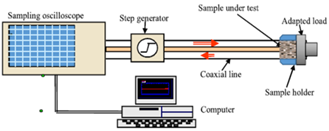Figure 1. The instrumentation used in temporal spectroscopy

3.2 Measurement and parameters extraction procedure

In this measurement stage, the experimental method chosen deals with multiple reflections of the adapted line whose configuration is shown in Figure 1. This experiment consists of time signal processing representing the TDR system dielectric response for the sample under test, and acquired from the digital sampling oscilloscope. The first parameter to be extracted from the measurement data on which all calculations are performed is the reflection coefficient $\Gamma(\omega)$. This is deduced from the measurements of the reflected V-(t) and the incident V+(t) signals by applying the Fourier transform and which is noted in the frequency domain as follows:

$\Gamma(\omega)=\frac{V^{-}(\omega)}{V^{+}(\omega)}$      (5)

where, V+(w) and V-(w) are derived from the measured time signals V+(t) and V-(t) of the sample.

The input admittance can be derived from the reflection coefficient as follows:

$Y_{i n}(\omega)=\frac{1-\Gamma(\omega)}{1+\Gamma(\omega)}$      (6)

From this Eq. (6), we have established a relation between the input admittance Yin(w), the characteristic admittance Y0 and the complex dielectric permittivity ε and it is written down as transcendental equation which is expressed as follows:

$\frac{Y_{i n}(\omega)}{Y_{0}}=\frac{\sqrt{\varepsilon^{*}} \cdot \tanh \left(j \cdot \frac{\omega d}{c} \cdot \sqrt{\varepsilon^{*}}\right)+1}{1+\frac{\tanh \left(j \cdot \frac{\omega d}{c} \cdot \sqrt{\varepsilon^{*}}\right)}{\sqrt{\varepsilon^{*}}}}$     (7)

A numerical method  made it possible to find several solutions to solve Eq. (7) in a complex plane and thus to obtain a final value of the complex permittivity.

According to Debye's model, considered to be frequently used for a wide frequency range, the complex dielectric permittivity can be given by:

$\varepsilon^{*}=\varepsilon^{\prime}-j \varepsilon^{\prime \prime}=\varepsilon_{\infty}+\frac{\varepsilon_{s}-\varepsilon_{\infty}}{1+j \omega \tau}-j \frac{\sigma_{s}}{\omega \varepsilon_{0}}$      (8)

From this equation, we can note that the third term $\varepsilon^{\prime \prime}$ is considered as an ohmic contribution of the dielectric permittivity at low frequency (the presence of the parameter $\sigma_{S}$ which expresses the static conductivity of the material) and it could be written as follows:

$\varepsilon^{\prime \prime}=\frac{\sigma_{s}}{\omega \cdot \varepsilon_{0}}$      (9)

3.3 Sample preparation

The prepared samples are three materials ternary combinations types: epoxy resin (RE), one of the three titanates (MT, CT, BT) and manganese dioxide (MnO2). These were used in our previous research work for dielectric constant improvement . In the present work, the re-use of these composites is aiming to the new equivalent binary model approach validation. They are produced using a manufacturing protocol allowing us to have composite materials with reproducible dielectric characteristics. This epoxy resin component is used as a matrix acting as a binder for two additional loads. One of these is a titanate chosen from a set of three (MT, CT, BT) each representing the second constituent of the mixture while the included second load is manganese dioxide (MnO2). Each sample is composed of 70% epoxy resin and the loads proportions jointly constitute 30% of the mixture and their constituents vary complementarily in steps of 7.5% (0%, 7.5%, 15%, 22.5% and 30%). The 70% fixed concentration level of epoxy resin is considered as the minimum volume fraction that can lead to samples without shrinkage after polymerization and with a better densification. These mixtures are made at room temperature and under atmospheric pressure. After the hardening process, the materials are machined in a way to adjust the samples to the measuring cell dimensions of the coaxial guide used in the TDR measuring bench. These samples are of toroid shape compatible with the used APC -7 mm standard coaxial line.

4. Results and Discussion

4.1 Ternary composites: Dielectric permittivity determination

## 2.pngFigure 2. The dielectric permittivity real part evolution as a function of the titanates volume fraction

Figure 2 shows the variation of the dielectric permittivity of the composite based on epoxy resin (RE), one of the three titanates (BT, CT, MT) and manganese dioxide (MnO2). From the graphical results examination, one can note a visible increase in the dielectric permittivity of the composite ($\varepsilon^{\prime}$) while the volume fraction of MnO2 is upward. The highest value estimated at 9.606 is reached for a 30% MnO2 concentration to which the composite is rather binary. This resulting dielectric parameter increase is probably due to the titanate behavior which is more conductive than that of MnO2. The three curves converge towards the same point where the three materials free of titanates correspond to RE-MnO2 binary mixtures.

4.1.1 Evaluation of the ternary composite shape factor

In the modeling process based on the MLL, it is essential to research the shape factor attributed to each of the ternary composites models. This is introduced to achieve a better optimization of the composite dielectric behavior predictive model and to fall upon a good agreement between the results of both theoretical model and experiments. The measured values of this factor (Figure 3) show a certain dependence on the volume fraction of titanates or manganese dioxide due to the mixture particle size random nature, and thus leading to a $\varepsilon^{\prime}$ values set which corresponds to the samples series under the measurement process. It can be seen from the figure that the trend of the curve of RE-MT-MnO2 is different from that of the other two composite materials. This discrepancy could probably be due to their different crystalline structures which are ilemnit and perovskite respectively.

## 3.png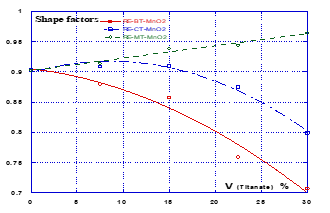Figure 3. The different composites shape factors values

## 4.pngFigure 4. The shape factors values estimated for the different composites using the three types of approximation

Thereafter, mathematical optimization approaches of this MLL based predictive model have undergone a better smoothing of this shape factor and hence close-fitting between the theoretical and experimental results. These mathematical methods being involved here consist of the shape factor estimation using three types of approximation: an average value (Favg), a linear regression (Flin) or a polynomial smoothing (Fpol) approximations (Figure 4). The last two approximations are implemented in the Kaleida-graph software that provides best polynomial order and coefficients with a minimum error between experimental and theoretical data.

Figure 5 shows the results of comparison made between the dielectric permittivity values using the modified Lichtenecker law predictive model and those found by the time domain spectroscopy measurement method. According to this figure graph, one can see the closeness of the composites dielectric permittivity curves and from which it comes out that these curves obtained by the linear (in RE-MT-MnO2 case) and polynomial (in RE-BT-MnO2 and RE-CT-MnO2 cases) approximations of shape factors are in good agreement with the experimental ones. However, the shape factor approximated to an average value provides relatively substantial deviations from the experimental second case curves found experimentally, thus making this approach impractical. From the view point of the titanate type influence on the composite permittivity, it is noticed graphically that the RE-MT composite provides a tendency curve different to those obtained for both BT and CT based composites. This discrepancy is pointed out by the curve concavity change nevertheless keeping all the curves with a dielectric permittivity decrease rate variation in terms of the upward titanate volume fractions. This behavioral change could be a consequence of the crystalline structure difference of both the BT/CT titanates and the MT titanate which are perovskite-type and ilmenite-type structures respectively.

## 51.png(a) Composite (RE - BT - MnO2)

## 52.png(b) Composite (RE - CT - MnO2)

## 53.png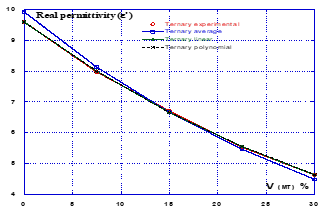(c) Composite (RE - MT - MnO2)

Figure 5. Comparison between the theoretically predicted values using the three approximations and those found experimentally

Moreover, the credibility of this comparative study should get through an estimate agreement between the experimental and theoretical values. To this goal achievement, one of the means used to quantify their closeness/divergence is to evaluate the real permittivity relative error by using this optimization method. This error is quantified as the ratio of the difference between the experimental and theoretical values of this permittivity over the practical value of the latter and which is expressed as follows:

$\left.Q(Y \operatorname{com} p)\right|_{\%} ^{F a}=\frac{\left|\varepsilon_{e x p}^{\prime}-\varepsilon_{F a}^{\prime}\right|}{\varepsilon_{F a}^{\prime}} \times 100$       (10)

The error quantity values are arranged in Table 1 regarding their corresponding composite volume fractions (XT), the mixture nature and the approximation type (AT) applied to the shape factor estimation. The obtained relative errors undergo fluctuations which are probably due to imperfections accumulated during the samples preparation phase, or to systematic and hazardous errors originating from the measuring bench. Besides, the use of linear and polynomial approximations have conducted to more accurate results comparing to the average value approximation and hence improving much more the performance of this MLL model. According to the table results, the best score of the error doesn’t exceed 1% and was recorded by use of the polynomial approach for all titanate concentrations over all composites, excepting the two barium titanate concentrations (15% and 22.5%), where this inaccuracy increased and reaching 3%. So, we can conclude that both predictive models and experimental results are in good agreement and the modified Lichtenecker model remains relevant with a tolerable uncertainty margin for ternary composites. This leads us to confirm once again the modified Lichtenecker law validity for an efficient prediction of the ternary mixtures dielectric behavior.

4.1.2 Numerical study of the MnO2 effect on the ternary composite model

The aim of this study is to investigate the effect of the MnO2 on the models of three different titanates composites with several fixed resin epoxy concentrations (from 70% to 95% with a 5% step). Figure 6.a represents the effective permittivity real part variation of the ternary mixture RE-BT-MnO2 as a function of manganese dioxide volume fraction over a range from 0 to 30% for different fixed values of either barium titanate (BT) concentration or Epoxy-resin matrix (RE) volume fraction. From the graphical results examination, and in BT fixed concentration value case it can be noted that the $\varepsilon^{\prime}$ evolution of the mixture leads to a non linear curves family delimited by both 0% and 30% of BT concentration values curves giving respectively maximum and minimum values of the dielectric constant. It clearly appears that any increase in the barium titanate concentration leads to a decrease in the dielectric constant. The maximum value of $\varepsilon^{\prime}$ is inversely proportional to the resin concentration. On the other hand, the dielectric constant $\varepsilon^{\prime}$ exhibits a growing linear curves family in the upward direction of manganese dioxide (MnO2) volume fraction while observing the RE fixed concentration values case. For the two other composites of Figures 6.b and 6.c using calcium titanate (CT) and magnesium titanate (MT) respectively, we report the same observation as that established for the first composite.

## 61.png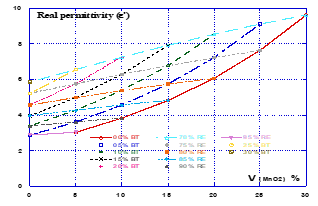(a) Composite (RE - BT - MnO2)

## 62.png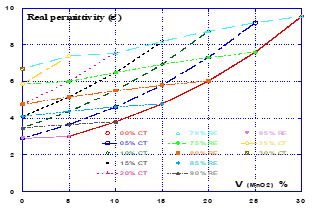(b) Composite (RE - CT - MnO2)

## 63.png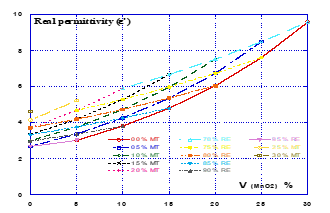(c) Composite (RE - MT - MnO2)

Figure 6. The permittivity variation as a function of manganese dioxide (MnO2) volume fraction (RE = const., Titanate = const.)

Table 1. The real permittivity relative error obtained using the three approximations

 XT (%) $\left.Q(Y \operatorname{comp})\right|_{\%} ^{F a}$ RE-BT-MnO2 $\left.Q(Y \operatorname{comp})\right|_{\%} ^{F a}$ RE–CT-MnO2 $\left.Q(Y \operatorname{comp})\right|_{\%} ^{F a}$ RE–MT-MnO2 AT Favg Flin Fpol Favg Flin Fpol Favg Flin Fpol 0 9.9 2.2 0.2 2.7 2.6 0.4 3.2 0.1 0.1 7.5 7.0 0.7 0.3 3.4 0.6 0.9 2.0 0.3 0.3 15 4.2 4.2 1.9 3.4 3.4 0.2 0.6 0.6 0.5 22.5 7.5 1.4 2.6 0.5 2.3 0.6 1.2 0.4 0.4 30 13.8 1.6 1.0 9.0 3.7 0.3 3.3 0.1 0.1

Three other important points that can be drawn from these graphs are:

1- The composite iso-curves families corresponding each to a fixed parameter either the resin (RE) or the titanate (CT, BT or MT) follow the same trend characterized by the composite effective permittivity increase in terms of increasing MnO2 concentration: this clearly explains the positive contribution in terms of dielectric behavior.

2- The iso-curves families for each composite show two different dielectric behaviors due to the type of considered fixed constituent parameter. For each constant resin concentration, the composite dielectric permittivity varies linearly for increasing oxide inclusion. While it is rather nonlinear if this fixed parameter is titanate: this behavior is perhaps explained by the different nature of structure and physics of these two constituents, resin and titanate.

3- In contrast to the spaced iso-curves for composites based on BT and CT, the MT based composite presents increasingly less spaced curves for titanate fixed concentrations. On the other hand, linearity slopes of this composite curves case are more abrupt than those presented by the two previous ones for the constant resin concentrations case: this latter composite behavior can be explained by a quick dielectric saturation phenomenon which may be is due to its disposal of an ilmenite structure different to the perovskite one of the other composites.

4.2 The new binaries: the proposed model validation

In this part of the work, the main attention is given to the ternary composite description as a new equivalent binary composite by modeling means and whose constituents namely resin, titanate and oxide remain unchanged. The goal to be achieved is twofold: it is time and cost savings. For example, manufacturing a composite with n components (n greater than 3) for better dielectric performance can require a great deal of stoichiometric effort and precise measurement of all the components used as well as their dielectric permittivity knowledge. To this purpose, several necessary tests and measurements will require then a great deal of specific materials and equipment, consequently involving relatively large enough time consumption, in order to obtain satisfactory results. There is an urgent need to solve this problem by establishing models which predict the dielectric behavior of this composite type with a certain acceptable accuracy degree. This has been already proved by the modified Lichtenecker model for ternaries and even for quaternaries [14, 15]. However, if the constituents number n increases as high as possible (n greater than 4), it will make the MLL model complex whose parameters number increases proportionally with that of mixture components. In addition, some of these elements were combined to each other in binary, ternary or other composites for specific applications and they have already dielectric permittivity validated theoretically and experimentally (using time domain reflectometry). It would be very important to use this resulting composite parameter instead of individual component permittivity if these composite constituents are a part of a complex mixture: the parameter size of the modeling expression will be reduced by n-2.

The new binary model to be constructed has the ternary composite as one of its elements and whose real permittivity values are already determined from both experiments and the modified Lichtenecker's law. This element is considered in this new mixture as a filler bathed in Resin-Epoxy (matrix) as the second mixture component giving rise to a new equivalent and simplified binary composite namely Re-(Re-XT-MnO2). In modeling point of view, and whose approach will be described in the following section, this attempt aims to simplify the ternary mixture model into a binary mixture model. This makes it possible to reduce the predictive law parameters and making this law simpler and easier to process.

4.2.1 Binary model design approach

The basic idea of a binary model equivalent to the ternary mixture is to develop a numerical method based on the modified Lichtenecker law to model these ternary composites in an equivalent and simplified binary mixture model. This new equivalent binary mixture uses as constituents Resin-Epoxy and one of the ternary composites mentioned above. This constituent is taken as a powder obtained after grinding and whose grains have a random morphology representing 30%, 20% or 10% of the mixture overall volume. The remaining volume concentration, being 70%, 80%, or 90% respectively is that of the resin in which the composite is immersed. These stoichiometric quantities were observed on the instructions basis to take care with in order to avoid the viscosity problem encountered with mixtures prepared in previous works. The real permittivities of these two constituents are known beforehand and whose values are obtained by means of the experimental measurement bench based on the TDR and from the modified Lichtenecker model in the ternary composite case. The resin real permittivity value is obtained for a mixture made of resin only. We point out that the stoichiometric compositions of resin and ternary composite remain unchanged throughout each step of simulation process. However, the ternary composite real permittivity changes because of its dependency on the titanate or oxide concentration level contained therein. These last two elements (titanate and oxide) are complementary in their ternary mixture where they represent 30% of the total volume and in which they vary between themselves at a fixed step of 7.5%. This new equivalent binary model remains predictive whose accuracy depends on that of the modified Lichtenecker model which has proved its efficiency in previous works. It also uses this same law for its real permittivity evaluation and is expressed mathematically as:

$\varepsilon_{B M}^{\prime}=F_{B} \times\left(\varepsilon_{R e}^{\prime}\right)^{f} \times\left(\varepsilon_{R e X t O x}^{\prime}\right)^{1-f}$      (11)

The elements Re, XT and Ox are the primary resin, the titanate (barium, calcium or magnesium titanate) and manganese dioxide (MnO2) respectively, f is the secondary resin volume fraction set at either 70%, 80% or 90%. FB is the new equivalent binary mixture shape factor.

The permittivity value of this new binary composite ε’BM must be that of the ternary composite which has been deduced according to Eq. (12) from the MLL model of the ternary mixture or experimentally and we noted as:

$\varepsilon_{B M}^{\prime}=A \times\left(\varepsilon_{R e}^{\prime}\right)^{70 \%} \times\left(\varepsilon_{X T}^{\prime}\right)^{x}\left(\varepsilon_{O x}^{\prime}\right)^{y}=\varepsilon_{\exp }^{\prime}(\operatorname{ReXTO} x)$      (12)

with x + y = 30%.

$A, \varepsilon_{\exp }^{\prime}(\operatorname{ReXTOx}), \varepsilon_{R e}^{\prime}, \varepsilon_{X T}^{\prime}$ and $\varepsilon_{O x}^{\prime}$ are the shape factor, the ternary composite (ReXTOx), the resin, titanate and oxide real permittivities respectively whose values are given in the previous sections.

4.2.2 The shape factor evaluation and the new binary model optimization

The shape factor FB evaluation is introduced in order to give with some probabilities an idea on the powder grains shape of the new composite included in the resin and which altogether constitute a random mixture. By combining the two Eqns. (11) and (12), the values of the new shape factor are computed for three titanate (ReXTOx) cases such as the ReBTMnO2, ReCTMnO2 and ReMTMnO2, and recorded in Figure 7. We mention here that due to the composites and the matrix composition which are kept unchanged, different concentrations of the latter ranging from 70% to 90% were carried out however without affecting the mixture viscosity. The interest sought here is to see if the resin volume variation can affect the shape and trend of the dielectric permittivity curve of this simulated composite.

## 71.png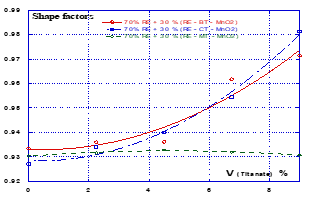(a) Composite (70% RE + 30% ternary composite)

## 72.png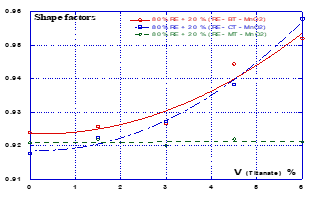(b) Composite (80% RE + 20% ternary composite)

## 73.png(c) Composite (90% RE + 10% ternary composite)

Figure 7. The shape factors values for the different binary composites

Then, numerical optimization approaches of this predictive MLL based model undergo better shape factor smoothing in order to bring together ternary composites and its equivalent binary ones permittivity values closer and thus providing a best possible precision.

This accurate shape factor evaluation calls on the previous numerical method being performed according to one of the three following approximation types: an average value (Favg), a linear smoothing according to the linear regression method (Flin(x) = M1.x + M0) or polynomial smoothing (Fpol(x) = M2.x2 + M1.x + M0) (Figure 8).

## 81.png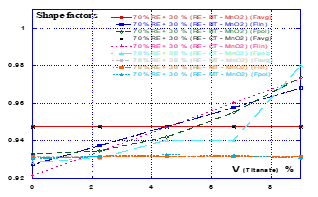(a) Composite (70% RE + 30% ternary composite)

## 82.png(b) Composite (80% RE + 20% ternary composite)

## 83.png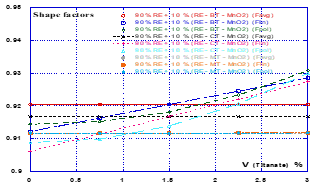(c) Composite (90% RE + 10% ternary composite)

Figure 8. The shape factors values for the different composites using the three approximation types

Our proposed model precision and its validation must ultimately pass through two comparative studies, one of which allows the selection of the shape factor approximation approach producing the minimum dielectric permittivity error amount possible. While the second comparison concerns the MLL model closeness degree to the experimental values and to those provided by the other theoretical models mentioned previously as well. From Figures 9, 10 and 11, it appears clearly that the MLL model obtained according to the proposed approach for the binary ones provides permittivity values which follow in good agreement those found for the ternaries.

## 91.png(a) Composite (RE - BT - MnO2)

## 92.png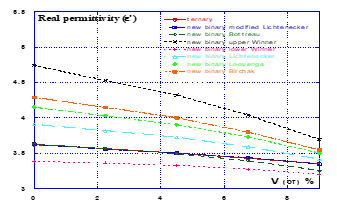(b) Composite (RE - CT - MnO2)

## 93.png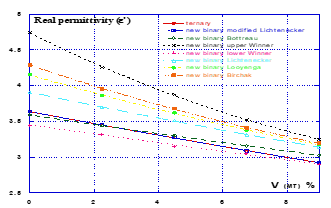(c) Composite (RE - MT - MnO2)

Figure 9. The permittivity evolution for binary composites (70% RE + 30% ternary composite)

Moreover, Bottreau's law (Bot) which is considered to be very suitable for binaries has also given satisfactory results. This law has been introduced to solve the problem being faced with the classical Lichtenecker law that does not give a convincing answer when dealing with non spherical granular structure . It consists of representing graphically the dielectric permittivity as load and matrice volume fractions function in a logarithmic scale,using the following expression:

$\ln (\varepsilon)=p_{1} \ln \left(\varepsilon_{1}\right)+p_{2} \ln \left(\varepsilon_{2}\right)$      (13)

$p_{1}$ and $p_{2}$ are the constituents probabilities, given by these formulas:

$p_{1}=\frac{\left(\frac{V_{1}}{1-V_{i}}\right)^{\alpha}}{\left(\frac{V_{2}}{V_{i}}\right)^{\alpha}+\left(\frac{V_{1}}{1-V_{i}}\right)^{\alpha}}$      (14)

and:

$p_{2}=\frac{\left(\frac{V_{2}}{V_{i}}\right)^{\alpha}}{\left(\frac{V_{2}}{V_{i}}\right)^{\alpha}+\left(\frac{V_{1}}{1-V_{i}}\right)^{\alpha}}$      (15)

## 101.png(a) Composite (RE - BT - MnO2)

## 102.png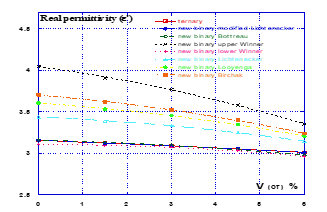(b) Composite (RE - CT - MnO2)

## 103.png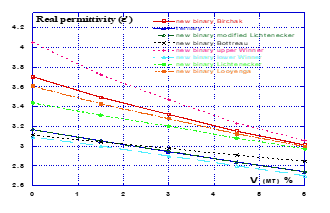(c) Composite (RE - MT - MnO2)

Figure 10. The permittivity evolution for binary composites (80% RE + 20% ternary composite)

Bottreau's law showed the best agreement between the theoretical and experimental results for binary composites and this result from the Bottreau distribution parameters (Vi, a) which made it possible to bring the theoretical model closer to the experimental results. The Bottreau parameters are given in Table 2.

Table 2. The parameters of Bottreau's law

 Bottreau parameters 70% RE + 30% ternary 80% RE + 20% ternary 90% RE + 10% ternary RE + ternary (RE - BT - MnO2) Vi 0.15 0.10 0.05 α 0.43 0.51 0.70 P1 0.25 0.14 0.03 P2 0.75 0.86 0.97 RE + ternary (RE – CT – MnO2)) Vi 0.15 0.10 0.05 α 0.44 0.52 0.74 P1 0.24 0.13 0.02 P2 0.76 0.87 0.98 RE + ternary (RE – MT – MnO2) Vi 0.15 0.10 0.05 α 0.46 0.55 0.80 P1 0.23 0.12 0.01 P2 0.77 0.88 0.99

## 111.png(a) Composite (RE - BT - MnO2)

## 112.png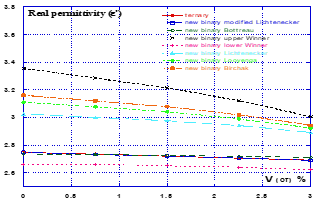(b) Composite (RE - CT - MnO2)

## 113.png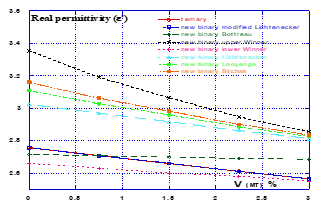(c) Composite (RE - MT - MnO2)

Figure 11. The permittivity evolution for binary composites (90% RE + 10% ternary composite)

Regarding the use of conventional Lichtenecker, Looyenga and Birchak laws, it is aimed to measure how accurate our proposed and optimized model applied to this new binary equivalent composite is in comparison with these laws models. These are mostly applied to binary composites and they are mentioned as follow:

The conventional Lichtenecker law (CL) is given by the relation:

$\varepsilon=\varepsilon_{i}^{f} . \varepsilon_{m}^{(1-f)}$      (16)

The equation developed by Looyenga (Looy) is given by:

$\sqrt{\varepsilon_{e f f}}=f \sqrt{\varepsilon_{i}}+(1-f) \sqrt{\varepsilon_{m}}$      (17)

The equation developed by Birchak (Bir) can be written in the following form:

$\sqrt{\varepsilon_{e f f}}=f \sqrt{\varepsilon_{i}}+(1-f) \sqrt{\varepsilon_{m}}$      (18)

It is shown graphically from the forthcoming that these modeling laws being applied to the new binary composite produce a significant spacing in the permittivity values for all the titanate concentrations and overall ternary composites to those found experimentally. This confirms the proposed model performance. For our model validation purpose, the recourse to the upper and lower Winner laws is more than useful, since these allow us to drawing the lower and upper limits of the composites dielectric permittivity values in order to locate those of our model. Graphically, the permittivity limit values provided by Winner's laws are estimated using equations given underneath and really bordered our MLL model ones (Figures 9, 10, 11), and effectively this confirms the proposed model validity. The parallel (upper) and series (lower) Wiener's laws are given in Eqns. (3) and (4).

5. Conclusion

This paper presents a dielectric behavior study of various ternary composites as well as the simplification of ternary models into binary models using time domain spectroscopy (TDS) in the microwave domain. There are three examined composites, distinguished by their type of titanate, namely barium titanate (BT), calcium titanate (CT) and magnesium titanate (MT) titanates, each associated with manganese dioxide (MnO2) and epoxy resin as a binder. An important objective that emanates from this work is to develop not only a model describing the dielectric behavior of these composites in an optimal way but also to succeed in transforming at least numerically this model into a binary composite model having the same constituents. This task is obviously aimed at reducing the length of the mathematical expression describing this composite when dealing with a mixture of N constituents. Additionally, the proposed model validity is checked with those of the laws which are limited to binary mixtures and considered very competitive until now. The binary composites real permittivity values obtained using this model show a good agreement with those of the ternary composites obtained with both the measurement bench and this same MLL law model. This achievement has been justified by the low relative errors quantified from these results and that does not exceed 1%. Besides, the model optimization merit is due to the polynomial approximation approach applied to MLL model to both ternary and binary models. The used models could be of great help in predicting dielectric behavior of mixtures that can have applications in micro and nano-electronics and more particularly in the circuits miniaturization used in telecommunication and microwave electronics. It is projected in the near future to introduce artificial intelligence approach to better improve the dielectric behavior predictive model of heterogeneous multi-phase mixtures.

Nomenclature
 $\varepsilon_{0}$ the vacuum permittivity, F.m-1 $\sigma_{S}$ the static conductivity, Ω-1.m-1 $\boldsymbol{\tau}$ the relaxation time, s Greek symbol εeff the effective dielectric permittivity of the mixture εn the constituents dielectric constants αn the constituents volume fractions $\varepsilon_{S}$ the static permittivity $\varepsilon_{\infty}$ the high frequency permittivity ${\varepsilon}_{F a}^{\prime}$ the theoretical dielectric permittivities $\varepsilon_{e x p}^{\prime}$ the practical dielectric permittivities $\varepsilon_{B M}^{\prime}$ the real permittivities of the equivalent binary model $\varepsilon_{R e}^{\prime}$ the secondary resin real permittivities $\varepsilon_{R e X T O x}^{\prime}$ the ternary composite $\operatorname{ReXTOx}$ real permittivities $\varepsilon_{1}$ the ternary composite real permittivity (of volume fraction V1) $\varepsilon_{2}$ the resin real permittivity (of volume fraction V2) α the slope at the origin Subscripts pk depends on the mixture volume fraction. A the shape factor N the constituents total number $\omega$ the angular frequency d the sample thickness c the light speed in the vacuum Q(Ycomp) the relative error quantified as a percentage (%) for any composite X comp (i.e. titanate composite) Fa shape factor deriving from the MLL Vi the charge volume fraction at the inflection point
References

 Kyoung, R.H., Sojin, K., Hee J.K. (1998). New preparation method of low-temperature-sinterable perovskite 0.9Pb(Mg1/3Nb2/3)O3–0.1PbTiO3 powder and its dielectric properties. Journal of the American Ceramic Society, 81(11): 2998-3000. https://doi.org/10.1111/j.1151-2916.1998.tb02725.x

 Emelianov, N. (2015). Structure and dielectric properties of composite material based on surface-modified BaTiO3 nanoparticles in polystyrene. The European Physical Journal Applied Physics, 69(1): 1-5. https://doi.org/10.1051/epjap/2014140343

 Korotkova, T.N., Sysoev, O.I., Belov, P.A., Emelianov, N.A., Velyaev, Y.O., Al Mandalawi, W.M., Korotkov, L.N. (2016). Dielectric response of polystyrene – BaTiO3 nanocomposites. The European Physical Journal Applied Physics, 75(1): 1-4. https://doi.org/10.1051/epjap/2016160072

 Guerra, J.L.S., Bassora, L.A., Eiras, J.A. (2006). Microwave characterization of dielectric materials in the temperature range of 90-450 K. The European Physical Journal Applied Physics, 36(1): 65-70. https://doi.org/10.1051/epjap:2006105

 Zimmermang, F., Voigts, M., Weil, C., Jakoby, R., Wang, P., Menesklou, W., Ivers-Tiffée, E. (2001). Investigation of barium strontium titanate thick films for tunable phase shifters. Journal of the European Ceramic Society, 21(10-11): 2019-2023. https://doi.org/10.1016/S0955-2219(01)00164-9

 Mazet, L., Yang, S.M., Kalinin, S.V., Schamm-Chardon, S., Dubourdieu, C. (2015). A review of molecular beam epitaxy of ferroelectric BaTiO3 films on Si, Ge and GaAs substrates and their applications. Science and Technology of Advanced Materials, 16(3): 1-20. https://doi.org/ 10.1088/1468-6996/16/3/036005

 Brosseau, B., Beroual, A. (1999). Dielectric properties of periodic heterostructures: A computational electrostatics approach. The European Physical Journal Applied Physics, 6(1): 23-31. https://doi.org/10.1051/epjap:1999148

 Dinulović, M., Rašuo, B. (2001). Dielectric modeling of multiphase composites. Composite Structures, 93(12): 3209-3215. https://doi.org/10.1016/j.compstruct.2011.05.036

 El boubakraoui, M.C., Bri, S., Foshi, J. (2018). Dielectric Properties of SiCf/SiC Composites. FME Transactions, 46(1): 86-92. https://doi.org/10.5937/fmet1801086E

 Dinulović, M., Rašuo, B. (2009). Dielectric properties modeling of composite materials. FME Transactions, 37(3): 117-122.

 Myroshnychenko, V., Brosseau, C. (2005). Finite-element method for calculation of the effective permittivity of random inhomogeneous media. Physical Review E, 71(1): 1-16. https://doi.org/10.1103/PhysRevE.71.016701

 Jylhä, L., Sihvola, A.H. (2005). Numerical modeling of disordered mixture using pseudorandom simulations. IEEE Transaction Geoscience and Remote Sensing, 43(1): 59-64. https://doi.org/10.1109/TGRS.2004.839804

 Achour, M.E., Droussi, A., Lahjomri, F., Oueriagli, A. (2009). Modélisation des propriétés diélectriques des nanocomposites, Afrique Science, 5(1): 50-57. https://doi.org/10.4314/afsci.v5i1.61706

 Khouni, H., Bouzit, N., Martínez Jiménez, J.P., Bouamar, M. (2016). Study of dielectric behavior of ternary mixtures of epoxy/titanates (MgTiO3, CaTiO3, SrTiO3 and BaTiO3) with carbon black. The European Physical Journal Applied Physics, 76(2): 1-9. https://doi.org/10.1051/epjap/2016160305

 Bouchaour, M., Martínez Jiménez, J.P., Bouzit, N., Bourouba, N. (2018). Dielectric behavior of a quaternary composite (RE, BT, MnO2, CaO) in the band (DC–2 GHz). The European Physical Journal Applied Physics, 84(1): 1-10. https://doi.org/10.1051/epjap/2018180056

 Martin, J.C., Forniés-Marquina, J.M., Bottreau, A.M. (2003). Application of permittivity mixture laws to carbon black dielectric characterization by time domain reflectometry. Molecular Physics, 101(12): 1789-1793. https://doi.org/ 10.1080/0026897021000018367

 Sareni, B., Krähenbühl, L., Beroual, A., Brosseau, C. (1997). Effective dielectric constant of random composite materials. Journal of Applied Physics, 81(5): 2375-2383. https://doi.org/10.1063/1.364276

 Bounar, A., Bouzit, N., Bourouba, N. (2017). Electromagnetic characterization of a composite (RE-CB-MT) by time domain spectroscopy. Advances in Materials Science and Engineering, 2017(9): 1-8. https://doi.org/10.1155/2017/3571648